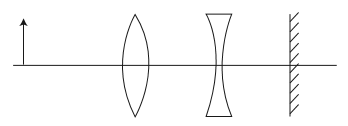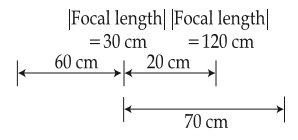#### A convex lens, of focal length 30 cm, a concave lens of focal length 120 cm, and a plane mirror are arranged as shown.  For an object kept at a distance of 60 cm from the convex lens, the final image, formed by the combination, is a real image, at a distance of :Option 1) 60 cm from the convex lens Option 2) 60 cm from the concave lens Option 3) 70 cm from the convex lens Option 4) 70 cm from the concave lens

As we learnt in

Thin lens formula -- whereinare object and image distance from lens.

Len's formula is given byFor convex lens =For concanve lens =Virtual object 10 cm behind plane mirror.

Hence real image 10 cm infront of mirror or 60 cm from convex lens.

Correct option is 1.

Option 1)

60 cm from the convex lens

This is the correct option.

Option 2)

60 cm from the concave lens

This is an incorrect option.

Option 3)

70 cm from the convex lens

This is an incorrect option.

Option 4)

70 cm from the concave lens

This is an incorrect option.

#### Vakul